In a previous article we showed the importance of using variables to replace multiple instances of the same measure in a DAX expression. A very common use case is that of the IF function. This article focuses on the cost for the formula engine rather than for the storage engine.

Consider the following measure.

```Margin :=
IF (
[Sales Amount] > 0 && [Total Cost] > 0,
[Sales Amount] - [Total Cost]
)
```

The basic idea is that the difference between Sales Amount and Total Cost should be evaluated only if both measures are greater than zero. When dealing with that condition, the DAX engine produces a query plan that evaluates each measure twice. This is visible in the storage engine requests generated for the following query.

```EVALUATE
SUMMARIZECOLUMNS (
'Date'[Year],
"Margin", [Margin]
)
```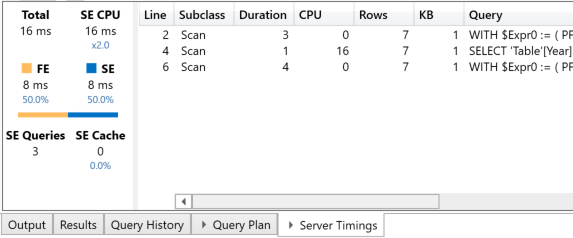However, it is worth stressing that the physical query plan has 216 rows, which is a reference point we will consider in later variations of the same measure.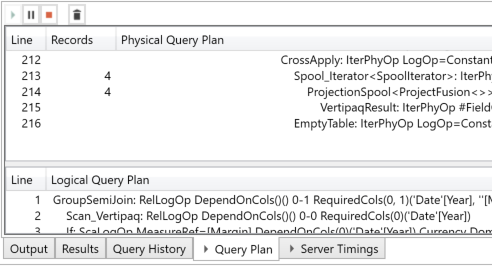Without going into details that were already explained in a previous article, it is worth noting that the multiple references to the same measure are requiring separate evaluations – although the result is the same. DAX is not the best at saving the value of common subexpressions evaluated in the same filter context. This is evident in the following variation of the Margin measure. The two branches of the IF function are identical, but the query plan adds other evaluations for both the storage engine and the formula engine.

```Margin 2 :=
IF (
[Sales Amount] > 0 && [Total Cost] > 0,
[Sales Amount] - [Total Cost],
[Sales Amount] - [Total Cost]
)
```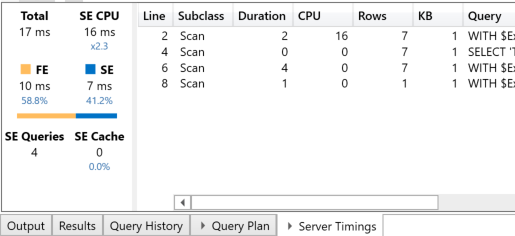In this case there is an additional storage engine query. The number of rows in the physical query plan is now 342. This increases the number of lines by over 50%, compared to the previous workload.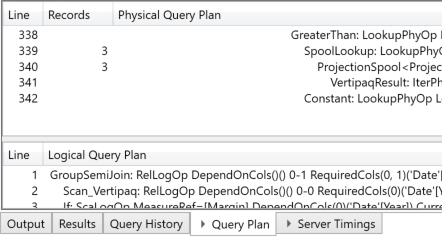The optimized version of this measure stores the two measures into two variables. This is so that they are only evaluated once in the IF function.

```Margin Optimized :=
VAR SalesAmount = [Sales Amount]
VAR TotalCost = [Total Cost]
RETURN
IF (
SalesAmount > 0 && TotalCost > 0,
SalesAmount – TotalCost
)
```

This is visible in the storage engine requests, of which there are only two.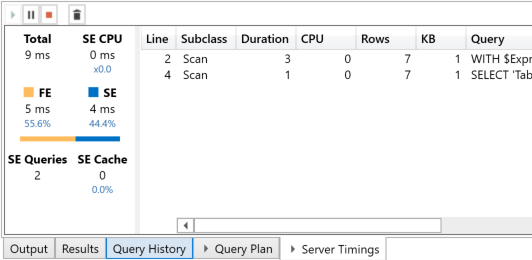A version of the IF function with the second branch identical to the first would produce the same storage engine queries.

The physical query plan reduced the number of rows from 216 to 126.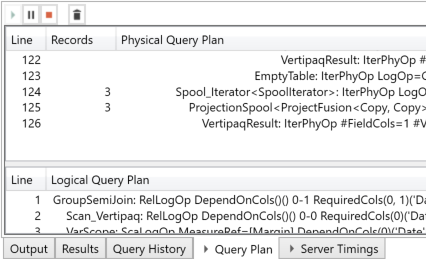This is an important result. This optimization technique is particularly useful when dealing with multiple references to a measure that has a high cost in the formula engine. Indeed, the DAX cache only operates at the storage engine level.

## Conclusion

Multiple references to the same measure in the same filter context can produce multiple executions of the same DAX expression, thus producing the same result. Saving the result of the measure in a variable generates a better query plan, improving code performance.

IFChecks whether a condition is met, and returns one value if TRUE, and another value if FALSE.

`IF ( <LogicalTest>, <ResultIfTrue> [, <ResultIfFalse>] )`